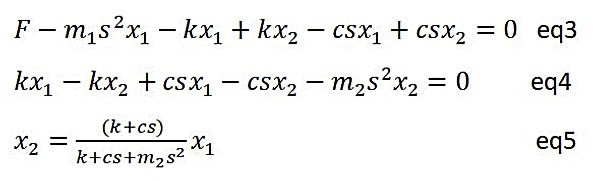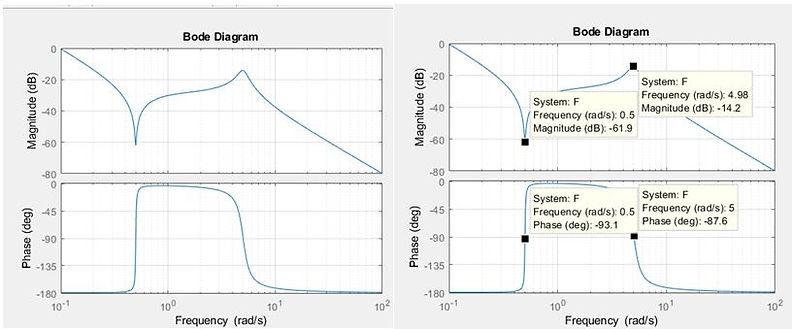naver-site-verification: naver47238a1b6bfbb19a2fd4b619734fa9a6.html
top of page

# The Bode diagram for double mass-spring system

Like in electrical engineering, Bode diagrams are widely used in mechatronics.

The Bode diagram program is explained on the control system pages so it is advised to study that page first.

The Bode diagram applied to the double mass spring system with damping will give insight into the behavior of the parameters. The results of the Ti calculations are compared with results obtained using Matlab. To apply the Bode diagram, a transfer function of the differential equations must be derivedTo plot the Bode diagram for X1 or/ X2 we have to transfer the differential equation into the s-domain which results in the following equations:Fill in Equation 5 in Equation 3 to obtain a transfer function for X1/F = H1 and X2/F = H2. These equations are ready to be entered into the Bode diagram program written for the TI-84 Plus.For Matlab, a special input file is written to plot the Bode diagram:

k=input('stiffness spring N/m = ')

c =input('damping Ns/m = ')

m1=input ('mass m1 kg = ')

m2=input ('mass m2 kg = ')

%numerator = [m2,c,k];

numerator = [m2,c,k];

denominator = [m1*m2,(m1+m2)*c, (m1+m2)*k,0,0];

F = tf(numerator,denominator);

bode(F);

The result is shown below  for m1=1kg, m2=100kg, c=1Nm/s, k=25 N/m## The Ti-84 Bodediagram

In the Ti program we use variable iX instead of variable S where X is the radian frequency (rad/sec).

Input:Output; explaining amplitude, phase and frequency:Green : Amplitude(dB), Brown: phase/10, Pink: frequency Rad/sec). The minimum is found @ -61.04038 dB (green), the belonging frequency  = 0.5 rad/sec (pink) and the phase is -9.05873 *10=-90.5873 degrees (Brown).

The minimum can be found with the command 2nd trace-minimum. With the ↑ ↓ keys, you can easily switch between the curves.

The maximum can be found with the command 2nd trace-minimum, which gives -14.15314dB @ 4,97 rad/sec with a phase of -8.44387*10=-84. 4387 degreesComparing the Ti-84 results with the MATLAB results show that the results match very well.

Interested in the Bode program BODEHOC.8XP ? Send a mail to frieboon@gmail.com

bottom of page